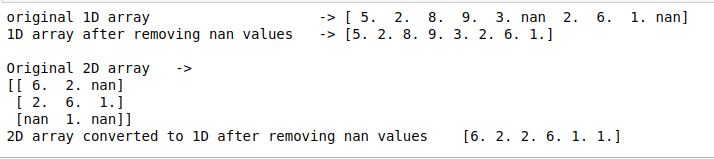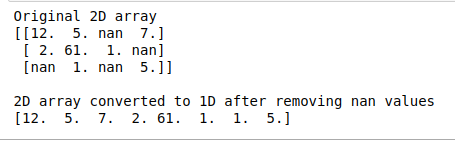# How to remove NaN values from a given NumPy array?

• Last Updated : 29 Aug, 2020

In this article, we are going to learn how to remove Nan values from a given array. man values are those values that do not have a specific value associated with it or they are different from the type of values that are to be used in the declared array.

There are basically two approaches that work in the same way, just a slight difference in syntax. Either we could use a function specified in NumPy or we could use an operator, the basic working will be the same.

Method #1 : Using numpy.logical_not() and numpy.nan() functions

The numpy.isnan() will give true indexes for all the indexes where the value is nan and when combined with numpy.logical_not() function the boolean values will be reversed. So, in the end, we get indexes for all the elements which are not nan. From the indexes, we can filter out the values that are not nan and save it in another array.

## Python3

 `import` `numpy`` ` ` ` `# create a 1D array``a ``=` `numpy.array([``5``, ``2``, ``8``, ``9``, ``3``, numpy.nan,``                 ``2``, ``6``, ``1``, numpy.nan])`` ` `# remove nan values using numpy.isnan()``# and numpy.logical_not``b ``=` `a[numpy.logical_not(numpy.isnan(a))]`` ` `# print the results``print``(``"original 1D array                    ->"``, a)``print``(``"1D array after removing nan values   ->"``, b)``print``()`` ` `# create a 2D array``c ``=` `numpy.array([[``6``, ``2``, numpy.nan], [``2``, ``6``, ``1``],``                 ``[numpy.nan, ``1``, numpy.nan]])`` ` `# remove nan values using numpy.isnan()``# and numpy.logical_not``d ``=` `c[numpy.logical_not(numpy.isnan(c))]`` ` `# print the results``print``(``"Original 2D array   ->"``)``print``(c)``print``(``"2D array converted to 1D after removing nan values   "``, d)`

Output:Note: No matter what Dimension of the array is, it will be flattened into a 1D array

Method #2 : Combining the ~ operator instead of numpy.logical_not() with numpy.isnan() function. This will work the same way as the above, it will convert any dimension array into 1D array.

In the below code only the 2D array is shown for example.

## Python3

 `import` `numpy`` ` ` ` `# create a 2D array``c ``=` `numpy.array([[``12``, ``5``, numpy.nan, ``7``], ``                 ``[``2``, ``61``, ``1``, numpy.nan],``                 ``[numpy.nan, ``1``,``                  ``numpy.nan, ``5``]])`` ` `# remove nan values using numpy.isnan() ``# and numpy.logical_not``d ``=` `c[~(numpy.isnan(c))]`` ` `# print the results``print``(``"Original 2D array   "``)``print``(c)``print``()`` ` `print``(``"2D array converted to 1D after removing nan values   "``)``print``(d)`

Output:My Personal Notes arrow_drop_up